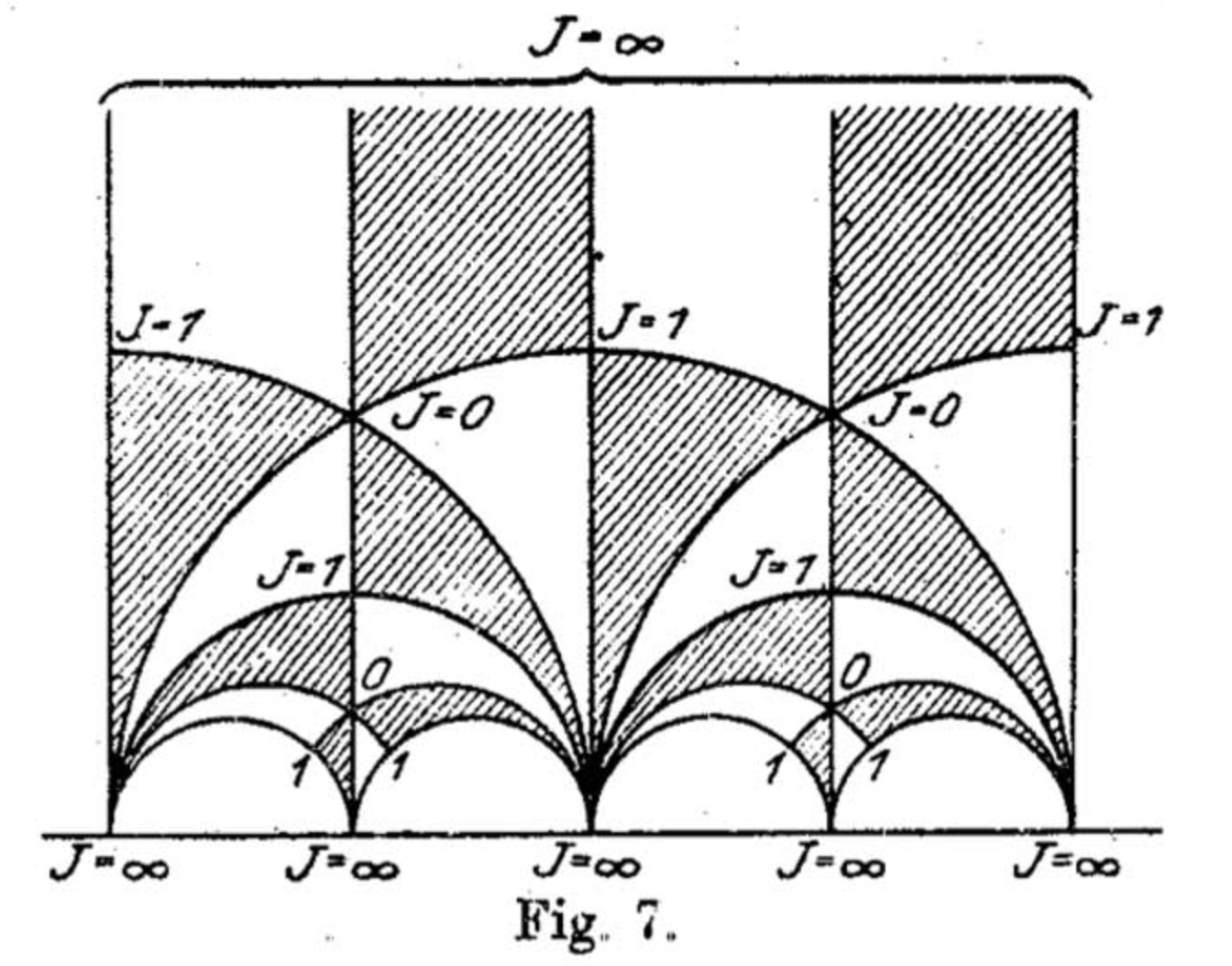# Origin of $j$-invariant

It is often asserted that the $$j$$-invariant was first introduced by Felix Klein. Is there any evidence for this claim? What works of Felix Klein do deal with it? What is the origin of the symbol $$j$$ used for it? Where it had first appeared?

## 1 Answer

Felix Klein, Über die Transformation der elliptischen Funktionen und die Auflösung der Gleichungen fünften Grades, Math. Ann. 14,‎ 111-172 (1878-1879).

There is also a slightly earlier brief note in Italian, Sull' equazioni dell' Icosaedro nella risoluzione delle equazioni del quinto grado, Rendiconti Reale Istituto Lombardo, Serie 2, vol. 10, p. 253-255‎ (1877).Dedekind may have gotten there first, see Dedekind or Klein (p. 67).

Notation: Klein used capital $$J$$, there is a factor 1728 difference with the $$j$$-invariant. (Read more about the origin of this factor.)